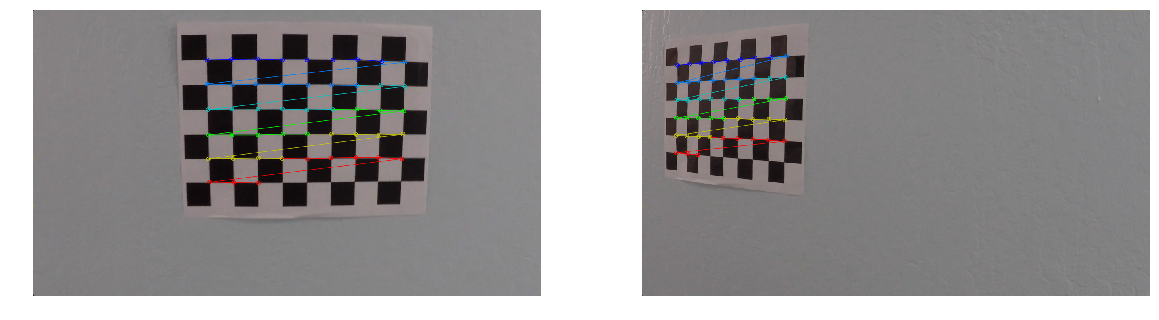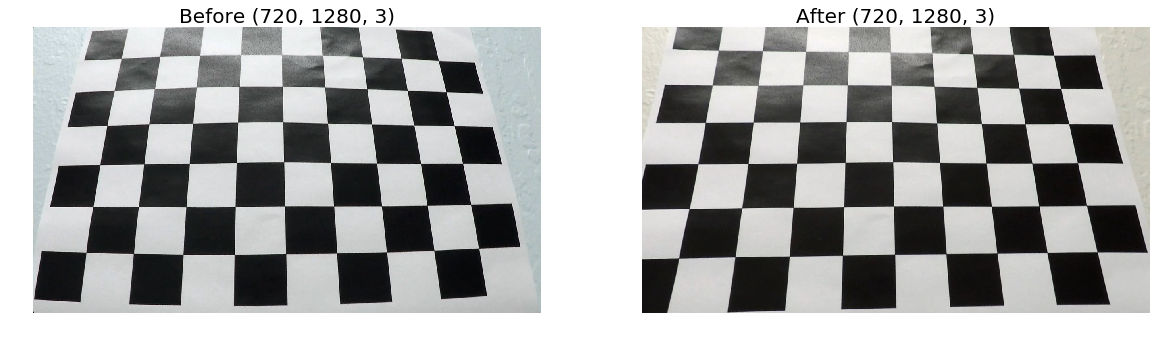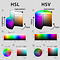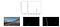# Goal

This is the report created for the fourth assignment of the first term of Udacity Self-Driving Car Engineer Nanodegree. The challenge was to create a improved lane finding algorithm, using computer vision techniques. The core of the work is a software pipeline that identifies the lane boundaries in a video from a front-facing camera on a car. The camera calibration images, test road images, and project videos were provided.

# Camera Calibration

When a camera captures 3D objects in the real world it transforms them into 2-dimensional images. This transformation isn’t exact and modifies the objects shape and sizes. In order to correct the distortion we need to analyze images generated with the camera, calibrate it and use the distortion parameters to correct other images taken with it.

## Chessboard images

The usual method to correct distortion is to calibrate the transformation using chessboard images. Python’s OpenCV library offers methods to address two common types of distortions:

• radial distortion (caused by the curvature of lenses)
• tangential distortion(caused when a camera’s lens is not aligned perfectly parallel to the imaging plane)Finding square intersections on the chessboard image to calculate coefficients

With OpenCV I calculated the camera matrix and distortion coefficients using chessboard images provided in the repository.

Then I used the calculated coefficients to undistort a chessboard image.Undistorting chessboard image

And did the same with a test image.

`def undistort_image(img, camera_matrix, distortion_coeffs):    img1 = cv2.undistort(img, camera_matrix, distortion_coeffs)    return img`

# Threshold Filters

After the correction for distortion is applied we need to run the image through an almost empirical combination of methods that process color channels and gradients in order to create a binary image that contains just the lane pixels. There is no exact formula so I had to incur in an iterative method that consisted of applying the filters with and verifying visually if pixels identified as part of the lane lines were, in fact, part of the lines. Here are some examples of these filters applied to the original test image.

This is where I spent most of the time dedicated to this project. Trying many combinations of filters with lots of different parameters to find the optimal results.

Some of the filters tried were:

• Along the X axis.
• Directional gradient with thresholds of 30 and 90 degrees.
• Magnitude gradient threshold.
• Red and green channel thresholds filters to detect yellow lanes

But I ended up just using color channel thresholds that achieved the goal.

• L (lightness) channel threshold eliminates edges generated from shadows in the frame.
• S (saturation) channel threshold enhances white & yellow lanes.
• H (hue) for the line colors.
`def color_thresh_combined(img, s_thresh, l_thresh, v_thresh, b_thresh):    V_binary = HSV_thresh(img, v_thresh)    S_binary = HLS_thresh(img, s_thresh)    L_binary = LUV_thresh(img, l_thresh)    color_binary = np.zeros_like(V_binary)                               color_binary[(V_binary == 1) & (S_binary == 1) & (L_binary == 1)] = 1return color_binary`Credit: subodh.malgonde, Udacity forum mentor– https://discussions.udacity.com/t/identifying-good-lanes/243822/18

# Bird’s-eye view

The next step in my pipeline is to warp the binary image (like the ones above) so it’s like it’s seen from above. That will allow the algorithm to fit a curve on the lane pixels as they were projected onto a 2D surface. Once the curve fit is done we can then unwarp the image back to the original perspective. Here are the test images.

# Finding the lines

Now that we have a thresholded image with a bird’s eye view the next step is to create an algorithm to identify left and right lane line pixels. And then fit these pixels with a 2nd degree polynomial, i.e. f(y)=Ay²​​+By+C.

## Histogram

To get a good indication of the base for the lane lines we add up pixel values along each pixel column in the binary image.

`import numpy as nphistogram = np.sum(img[img.shape//2:,:], axis=0)plt.plot(histogram)`

Since each pixel value is either 0 or 1 the two highest peaks in the histogram will likely be the x location for the base of the lane lines. This is where we should start to search for the lines.

Here is an example:Image and its pixel value histogram

## Sliding Window

From the base locations for both the left and right lines we create a sliding window–that’s located around the line centers– that finds and follow the lines up to the top of the frame.

Here are how some of the test images look after the sliding window method calculates the lane lines.

# Car position and lane curvature

Now that we have the polynomial fit for the lane lines we are able calculate the radius of curvature. As suggested in the course material I checked this reference for a tutorial on how to do it.

## Lane curvature

We say the curve and the circle osculate (which means “to kiss”), since the 2 curves have the same tangent and curvature at the point where they meet.

The radius of curvature of the curve at a particular point is defined as the radius of the approximating circle. This radius changes as we move along the curve.

The formula above was implemented in the function`radius_curvature` exemplified below.

`mean_curverad, position = radius_curvature(ploty, left_fitx, right_fitx, window_img.shape)The final curvature is the average for the left and right lane lines.`

NB: I used the assumption — as suggested in the course material and the forums — that:

• 30 meters is equivalent to 720 pixels in the vertical direction
• 3.7 meters is equal to 700 pixels in the horizontal direction.
`ym_per_pix = 30/720 # meters per pixel in y dimensionxm_per_pix = 3.7/700 # meters per pixel in x dimension`

## Car position/offset

The calculation for the car position — or rather, its offset from the center of the lane — used the following assumptions:

• The camera is positioned in the center of the car, or that its center is located in the center of the image.
• Same equivalence for meters and pixels as described above.

The center is calculated as the mean value between the bottom `x` of the left and right lane lines. The offset is the difference of the car center to the lane center.

NB: In the video annotation a negative value for the car offset mean it’s off to the left and a positive value means it’s off to the right. Obviously a null value means the car is exactly at the center of the lane.

# Lane boundaries

From this point to plot the lane boundaries we just needed to warp it back onto the original image. Some examples of the test images follow. The images contain an indication of the lane radius curvature and the car position in relation to the center of the lane.

# Video pipeline

Tying all these methods in sequence defines the final pipeline that processes each frame of the video. The pipeline below omits all parameters and returned variables for the sake of readability.

`def video_pipeline():    define_perspective_points()    undistort_image()    color_thresh_combined()    perspective_transformation()    find_lines_video()    invert_perspective()    superimpose_lane_area()`

This is the actual Python code for the video pipeline

`def video_pipeline(source_img):    src_pts, dst_pts = define_perspective_points(source_img)    source_img = undistort_image(source_img, mtx, dist)    thresh_img = color_thresh_combined(source_img, s_thresh, l_thresh, v_thresh, b_thresh)warp_img = perspective_transformation(thresh_img, src_pts, dst_pts, False)        left_fit_, right_fit_, lines_img, mean_curverad, position = find_lines_video(warp_img)inv_matrix, unwarp_img = invert_perspective(warp_img, src_pts, dst_pts)        lane_img = superimpose_lane_area(source_img, warp_img, left_fit_, right_fit_, inv_matrix, mean_curverad, position)    return lane_img`

# Final considerations and desired improvements

I tried a few iterations with the challenge videos but the shading and lighting conditions proved that I’d need to spend a more time refining the threshold calculation. I devised a way to output every frame so I iterated every frame that failed until I got optimal results. I would spend time trying different thresholding methods. My algorithm averages the lane calculation over the last N frames but still didn’t get the result I expected.

Multi time founder, CTO and product executive. Founder @ridehugobus, EIR: @human_ventures & @techstars. VC: @collabfund

## More from Alberto Escarlate

Multi time founder, CTO and product executive. Founder @ridehugobus, EIR: @human_ventures & @techstars. VC: @collabfund